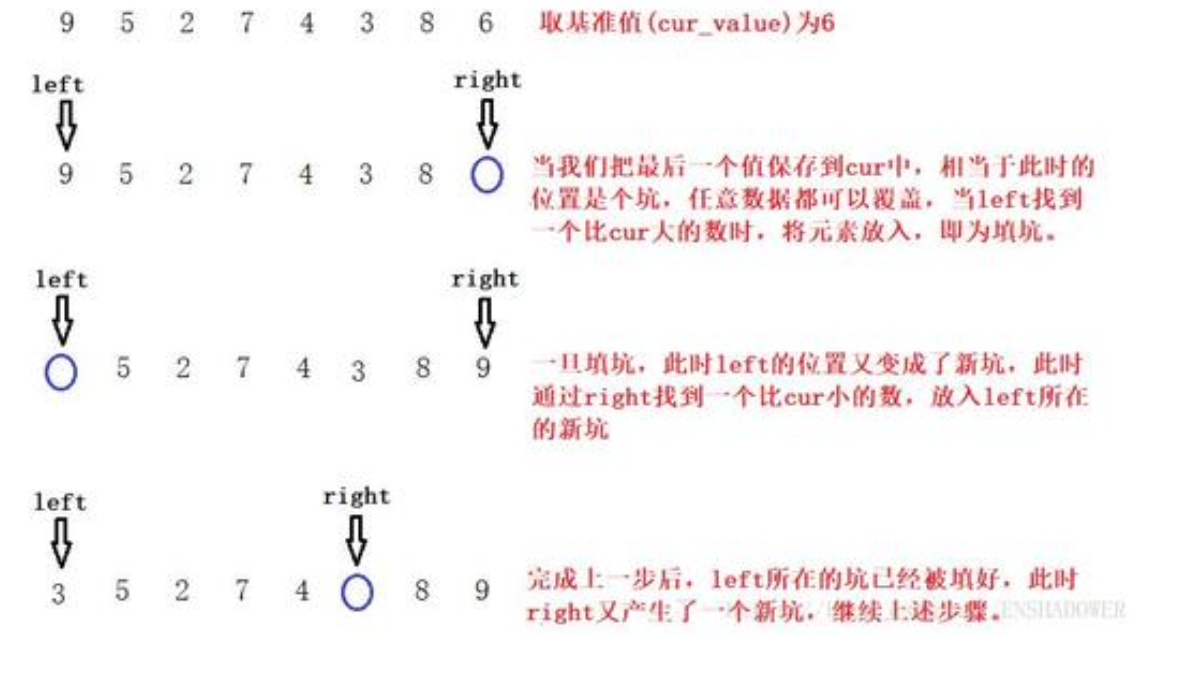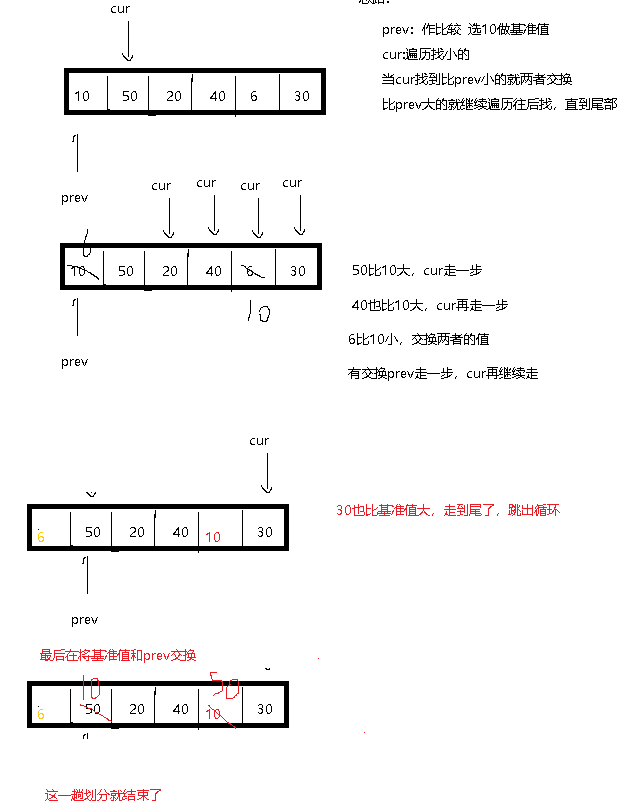# 快速排序 三种版本

19 篇文章 1 订阅

## 快速排序

1. 基础版本
2. 三数取中法
3. 挖坑法
4. 前后指针版本

1.直接选第一个元素做基准值
2.比较一趟 从最左边先开始，right 找小的，比基准值大的就往回走， left找大的，比基准值小了就往前走 两者停下，就交换一次，
3.再入循环 在重复以上步骤，
5. 最后一步，把基准值换到最后停下的下标，返回此下标索引

//普通版本
int PartSort(int *a, int left, int right)
{
int key = a[left];
int start = left;
while (left < right)
{
while (left < right && a[right]>=key)
{
right--;
}
while (left < right && a[left] <= key)
{
left++;
}
swap(&a[left], &a[right]);
}
swap(&a[left], &a[start]);
return  left;

}


1.先求left mid right这三下标的数据的中位数，return此下标 ，
2. 之后 放到第一个元素做基准值再套用基础模板

// 快速排序   三数取中优化法
int GitMidindex(int *a, int left, int right)//取三个数的中位数放到第一位做基准值
{
int mid = (left + right) / 2;
//int mid=left+ ((right-left)>>1) 可能整数溢出的处理方法
if (a[left] > a[mid])
{
if (a[mid] > a[right])
return mid;
else  if (a[right] > a[left])
return left;
else
return right;
}
else    //a[left] <a[mid]
{
if (a[mid] < a[right])
return mid;
else if (a[left]>a[right])
return left;
else
return right;
}
//返回中位数的索引
}
int PartSort1(int* a, int left, int right)
{
int mid = GitMidindex(a, left, right);//中位数的下标索引
swap(&a[mid], &a[left]);//与开头的那个值交换，确保最开始时是用中位数做基准值的
int key = a[left];
int start = left;
while (left < right)
{
while (left < right && a[right] >= key)
{
right--;
}
while (left < right && a[left] <= key)
{
left++;
}
swap(&a[left], &a[right]);
}
swap(&a[left], &a[start]);//比较完后把基准值换到停下的地方，一趟就排完了
return  left;

}


1.把选一个基准值先保存，再假想成一个坑，比较后满足条件的就把自己（覆盖）填过去，自己就又是坑了，再继续判断
2.直到填完只剩下最后一个空坑，把基准值放进去// 快速排序挖坑法
int PartSort2(int* a, int left, int right)
{
int key = a[left];    //把自己变成坑 a[left]就可以看成一个坑// 选左右都可以
while (left < right)
{
while (left<right && a[right]>=key)
{
right--;
}
a[left] = a[right];//小的就填进去  right就是新的坑了
while (left < right  && a[left] <= key)
{
left++;
}
a[right] = a[left];//大的填进去 left就是新的坑
}
a[left] = key;//最后再把基准值填到最后一个坑里
return left;//把中间的基准值的位置保留下来
}


prev指向开头，cur遍历找小，遇到小的便与之前prev保留的小的交换，遇到大的就继续遍历，这样小的一开始就交换到前面去了，大的不动直到最后被堆到后面。这样操作也就完成了划分以基准值为界的两段区间。// 快速排序前后指针法  双指针prev cur
int PartSort3(int* a, int left, int right)
{
int mid = GitMidindex(a, left, right);//选出中间的那个数
swap(&a[mid], &a[left]);//与开头的那个基准值交换，确保最开始时是用中位数做基准值的
int key = a[left];
int prev = left;
int cur = left + 1;
while (cur <= right)
{
if(a[cur] < key)
{
prev++;
swap(&a[cur], &a[prev]);
cur++;
}
else
{
cur++;
}
}
swap(&a[left], &a[prev]);
return prev;

}


void QuickSort(int* a, int left, int right)
{
//if (left >= right)//特殊情况  1 3 5 4， 当排第一趟时1为基准，end没找到比它小的一直减减，直到它本身了，此时left=right，返回keyindex为没动的left; 在递归下一趟时left为0，大于keyindex-1的-1，就不做左边无意义的递归趟数了
//	return;   如果区域不存在或只有一个数据则不递归排序
if (left < right)
{
if (left-right +1 < 10)//小区间优化：   区间内数据小于一定值了，就没必要用快排的递归下去了，小数据还是可以用插入排序的
{
InsertSort(a+left, right-left + 1); //相当于也是传区间
}
else
{//对不同版本调用即可
//int keyindex = PartSort(a, left, right);//基础版本
//int keyindex = PartSort1(a, left, right);//三数取中
//int keyindex = PartSort2(a, left, right);//挖坑法
int keyindex = PartSort3(a, left, right);//前后指针版本
// [left,keyindex-1]    keyindex   [keyindex+1,right]   二叉树结构  左区间 基准值 右区间  继续递归
QuickSort(a, left, keyindex - 1);//基准值左边（大于它的）继续划分
QuickSort(a, keyindex + 1, right);//基准值右边（小于它的）也继续
//直到最小的都已经有序（划分好了）
}
}
}

05-12
11-22662903-208万+
07-254万+
01-121万+
01-15532
03-14212
06-241万+
03-141467
07-23206
04-13739
11-2917万+
08-103万+
02-14120
12-12
03-176481
11-13429
04-201319
02-21991点击重新获取扫码支付余额充值